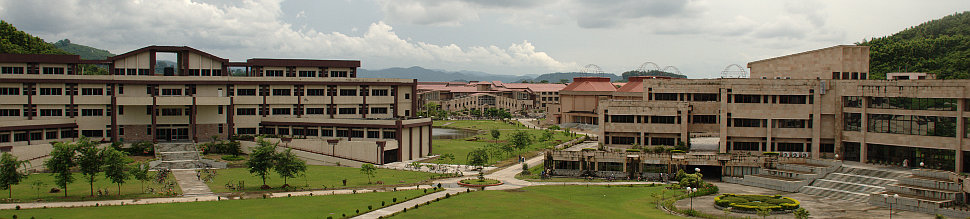••# Virtual Labs for Mechanical Vibrations(M)### Free Vibration of Spring-Mass system

To calculate the natural frequency and damping ratio of a spring-mass system, experimentally; and compare the results with theoretical values.### Free Vibration of a Cantilever Beam with a Lumped Mass at free end

To calculate the natural frequency and damping ratio for free vibration of a single DOF cantilever beam system, experimentally; and compare the results with theoretical values.### Forced Vibration of a Cantilever Beam with a Lumped Mass at Free End

To calculate the natural frequency and damping ratio for forced vibration of a single DOF cantilever beam system, experimentally; and compare the results with theoretical values.### Free Vibration of a Cantilever Beam (Continuous System)

To calculate the natural frequencies and damping ratio for free vibration of a cantilever beam considering as a continuous system, experimentally; and compare the results with theoretical values.### Forced Vibration of a Cantilever Beam (Continuous System)

To calculate the natural frequencies and damping ratio for forced vibration of a cantilever beam considering as a continuous system, experimentally; and compare the results with theoretical values.### Free Vibration of a Two-DOF System

To find the natural frequencies of a two degrees-of-freedom (DOF) system and the phase differences between the masses in the system in different modes of vibration.### .Free Vibration of a Viscously Damped Single DOF System

To analyze the free vibration response of a single DOF system at various damping conditions.### Harmonically Excited Forced Vibration of a Single DOF System

To analyze the forced vibration response of a single DOF system at different damping ratio and frequency ratio.### Harmonically Excited Rotating Unbalance of a Single DOF system

To analyze the forced vibration response of a single DOF system with rotating unbalance at different damping ratio and frequency ratio.### Harmonically Excited Support Motion of a Single DOF System

To analyze the response for forced vibration of a system excited by support motion at different damping ratio and frequency ratio.